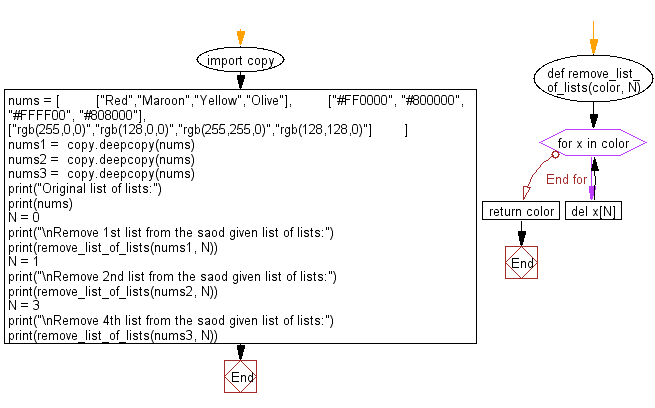﻿ Python: Remove the specific item from a given list of lists - w3resource# Python: Remove the specific item from a given list of lists

## Python List: Exercise - 140 with Solution

Write a Python program to remove the specific item from a given list of lists.

Sample Solution:

Python Code:

``````import copy
def remove_list_of_lists(color, N):
for x in color:
del x[N]
return color

nums = [
["Red","Maroon","Yellow","Olive"],
["#FF0000", "#800000", "#FFFF00", "#808000"],
["rgb(255,0,0)","rgb(128,0,0)","rgb(255,255,0)","rgb(128,128,0)"]
]
nums1 =  copy.deepcopy(nums)
nums2 =  copy.deepcopy(nums)
nums3 =  copy.deepcopy(nums)

print("Original list of lists:")
print(nums)
N = 0
print("\nRemove 1st item from the said list of lists:")
print(remove_list_of_lists(nums1, N))
N = 1
print("\nRemove 2nd item from the said list of lists:")
print(remove_list_of_lists(nums2, N))
N = 3
print("\nRemove 4th item from the said list of lists:")
print(remove_list_of_lists(nums3, N))
```
```

Sample Output:

```Original list of lists:
[['Red', 'Maroon', 'Yellow', 'Olive'], ['#FF0000', '#800000', '#FFFF00', '#808000'], ['rgb(255,0,0)', 'rgb(128,0,0)', 'rgb(255,255,0)', 'rgb(128,128,0)']]

Remove 1st item from the said list of lists:
[['Maroon', 'Yellow', 'Olive'], ['#800000', '#FFFF00', '#808000'], ['rgb(128,0,0)', 'rgb(255,255,0)', 'rgb(128,128,0)']]

Remove 2nd item from the said list of lists:
[['Red', 'Yellow', 'Olive'], ['#FF0000', '#FFFF00', '#808000'], ['rgb(255,0,0)', 'rgb(255,255,0)', 'rgb(128,128,0)']]

Remove 4th item from the said list of lists:
[['Red', 'Maroon', 'Yellow'], ['#FF0000', '#800000', '#FFFF00'], ['rgb(255,0,0)', 'rgb(128,0,0)', 'rgb(255,255,0)']]
```

Flowchart:## Visualize Python code execution:

The following tool visualize what the computer is doing step-by-step as it executes the said program:

Python Code Editor:

Have another way to solve this solution? Contribute your code (and comments) through Disqus.

What is the difficulty level of this exercise?

Test your Python skills with w3resource's quiz

﻿

## Python: Tips of the Day

Floor Division:

When we speak of division we normally mean (/) float division operator, this will give a precise result in float format with decimals.

For a rounded integer result there is (//) floor division operator in Python. Floor division will only give integer results that are round numbers.

```print(1000 // 300)
print(1000 / 300)```

Output:

```3
3.3333333333333335```